CBSE Worksheet-01

Class - IV Mathematics – Addition

Time Allowed: 30 minutes

1. Fill in the blanks with suitable words:
1. The addition of the numbers 172356, 242508 and 320531 is _________ .

2. The sum of numbers 100099, 45454, and 1230 is  _____.

3. The addition of numbers 730471 + 16435 + 2054 is  _____.

4. By adding number 13620 to the sum of numbers 14852 and 10001, we get the answer as

5. The addition of numbers 42184 and 32641 is  __________.

2. State whether each of the following statement is True or False:
1. In the addition of numbers 2346 and 6432, the digit at the hundreds place in their sum will be 7.

2. By adding the greatest 3 digit number to the sum of the greatest 4 digit number and greatest 5 digit number, we get the answer as 110997.

3. The sum of numbers 18642 + 11111 +  21331 is 51084.

4. When 0 is added to a number, the sum is the number itself.

5. When we add 360928 to the number 65035, the sum is 425963.

3. A bulb manufacturing factory produced 86325 bulbs in January, 69770 bulbs in February, and 107605 bulbs in March. How many bulbs did the factory produce all together in these three months?

1. 263800

2. 263600

3. 253700

4. 263700

4. When zero is added to a number, the sum is:-

1. One

2. The number itself

3. Zero

4. None of these

5. There are 267136 forks, 501243 spoons, and 789241 knives made in a factory. How many cutleries are made in total?

1. 15,57,602

2. 14,57,620

3. 15,75,620

4. 15,57,620

6. 2567853 + 999 = ________ + 2567853

1. 1000

2. 999

3. 998

4. 989

7. One less than 101001 is added to four more than 996. What will be the resultant number?

1. 101996

2. 103000

3. 102000

4. 102002

8. Match the following:

 (a) 334856 + 66666 (i) 200999 (b) 654309 + 1 (ii) 959255 (c) 775255 + 184000 (iii) 654310 (d) 190999 + 10000 (iv) 401522
9. Find the sum of 523456, 1211 and 234510.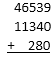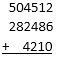11. Arrange in column and add

1. 176169 + 17178 + 18187
2. 316823 + 2322 + 451264
12. In a toy machine, 713082 dolls were made. Then 264529 teddy bears were also made by the same machine. How many toys were made in all by a toy machine?

13. In a factory, 57612 bikes were manufactured in the month of January and 26789 bikes in the month of February. How many bikes in total were manufactured in January and February?

14. Find the missing numbers:

1.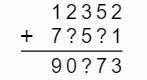2.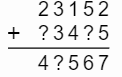3.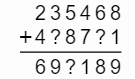4.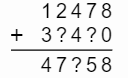15. On Sunday, 15896 men, 12458 women, and 20015 children visited the exhibition. How many people visited the exhibition in all that day?

1. In a stadium, there were 64000 spectators in the first show, 48000 spectators in the second show and 52500 in the last match. How many spectators came in the stadium in all the three matches
2. In a planetarium, there were 12505 children were there in the first show, 10275 students were there in the second show. How many children were present in both the shows.

CBSE Worksheet-01
Class - IV Mathematics – Addition

Solution

1. Fill in the blanks with suitable words:

1. 735395. By adding the digits at the ones, tens, hundreds, thousands, ten thousands and lakhs place, we get the answer.

2. 146783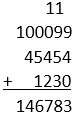3. 748960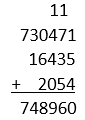4. 38473.  By adding 14852 and 10001, we get the sum as  24853. Now adding this sum to 13620, we get our answer 38473.

5. 74825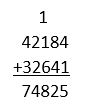2. State whether each of the following statement is True or False:

1. True. when we add 2346 and 6432, the sum we get is 8778.

2. True. 9999 + 99999 = 109998 + 999 = 110997.

3. True.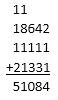4. True. 51 + 0 = 51

5. True. 360928 + 65035 = 425963

3. (d) 263700
Explanation: 86325 + 69770 + 107605 = 263700. They have asked us to find the total number of bulbs produced in 3 months. So we have to add the number of bulbs produced in January, February, and March to find the solution.
4. (b) The number itself
Explanation: Because 0 is the additive identity. That means when we add any number to 0, the sum will be number itself.
For example: 100 + 0 = 100 ; 5 + 0 = 5
5. (d) 15,57,620
Explanation: 15,57,620 is the correct answer.
Total cutleries = 267136 + 501243 + 789241 = 15,57,620
6. (b) 999
Explanation: 999 is the correct answer.
2567853 + 999 = _____ + 2567853 _____ = 2567853 + 999 - 2567853 _____ = 999
7. (c) 102000
Explanation: 102000 is the correct answer.
101000 + 1000 = 102000
8. (a) - (iv), (b) - (iii), (c) - (ii), (d) - (i)

9.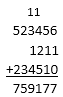10.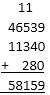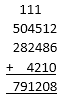1.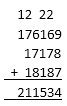2.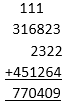11. Number of dolls made by the machine = 713082
Number of teddy bears made by the machine = 264529
Total number of toys made by the machine = 713082 + 264529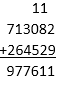The total number of toys made by the machine is 977611.

12. Number of bikes manufactured in the month of January = 57612
Number of bikes manufactured in the month of February = 26789
Total bikes manufactured = 57612 +26789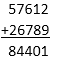Total bikes manufactured in the month of January and February is 84401.

1.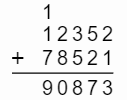2.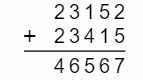3.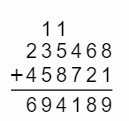4.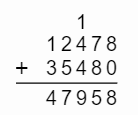13. Number of men = 15896
Number of women = 12458
Number of children = 20015
Total number of people = 12458 + 20015 + 20015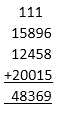48369 people visited the exhibition on Sunday.

1. Number of spectators in the first match = 64000
Number of spectators in the second match = 48000
Number of spectators in the third match = 52500
total number of spectators  = 64000 + 48000 + 52500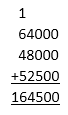164500 spectators came in all the three shows in the stadium
2. Number of children in the first show = 12505
Number of children in the second show = 10275
Total number of children = 12505 + 10275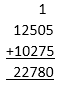22780 children were present in both the shows.